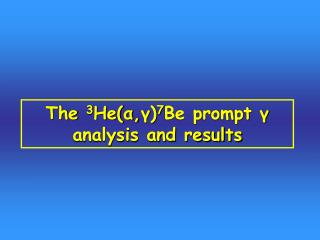DownloadDownload PresentationThe 3 He( α , γ ) 7 Be prompt γ analysis and results

# The 3 He( α , γ ) 7 Be prompt γ analysis and results

Télécharger la présentation## The 3 He( α , γ ) 7 Be prompt γ analysis and results

- - - - - - - - - - - - - - - - - - - - - - - - - - - E N D - - - - - - - - - - - - - - - - - - - - - - - - - - -
##### Presentation Transcript

1. The 3He(α,γ)7Be prompt γ analysis and results

2. Formulas

3. HPGe Efficiency Efficienza %

4. Summary

5. 400 keV (1) 1757 Experimental spectrum MC spectrum 1329

6. 400 keV (2) Experimental spectrum 429 MC spectrum

7. Angular distribution effects Krawinkel et al 1982 Angular distribution measurements were performed by Krawinkel et al at E> 350 keV At E< 350 keV only calculations are available: Parker-Kavanagh 1963 Kim-Izumoto-Nagatani 1981 a2~-0.2 @ E~200 keV for both primary transitions We changed the a2 coefficient from -0.1 to -0.3 and obtained 1.5% difference in the efficiency calculation

8. 400 keV Spectrum Ecm = 170.1 keV T = 4.35 d C = 112.7 C 429  0 Cts = 2584 DC  429 Eγ= 1327 keV Cts = 3332 DC  0 Eγ= 1756 keV Cts = 6773

9. 250 keV Spectrum Ecm = 106.1 keV T = 20.8 d C = 407 C 429  0 Cts = 508 DC  429 Eγ= 1263 keV Cts = 604 DC  0 Eγ= 1692 keV Cts = 1449

10. 220 keV Spectrum Ecm = 93.3 keV T = 30.1 d C = 637 C 429  0 Cts = 292 DC  429 Eγ= 1250 keV Cts = 345 DC  0 Eγ= 1679 keV Cts = 935

11. Systematic uncertainties ACTIVITY PROMPT-

12. σ (barn) Ecm (keV) Results (1)

13. Results (2) - red. syst. errors Fattore S (keV barn) Ecm (keV)

14. ALL LUNA RESULTS WITH TOTAL ERRORS

15. ALL LUNA RESULTS WITH TOTAL ERRORS (RED. SYST ERROR)

16. FOR EACH COUPLE (A-P) DISCREPANCY

17. FIT Simple minimization of 2 where the function is: F(E) = S(0)* f(E) and the Only fitting parameter is S(0). The error on S(0) is coming directly from the error on the fitting parameter. • Procedure (suggested by Filippo): • do the fit with statistical errors for prompt and activation separately • obtain statistical errors on fitting parameter S(0)_act and S(0)_prompt • ad quadratically red. Syst. Error • For final S(0): weighted average between S(0)_act and S(0)_prompt • For final error on S(0): add error from w.a. to common systematic error

18. FIT S(0) = 0.560  0.016 keV barn

19. DESCOUVEMONT KAJINO